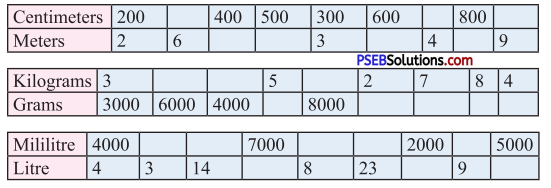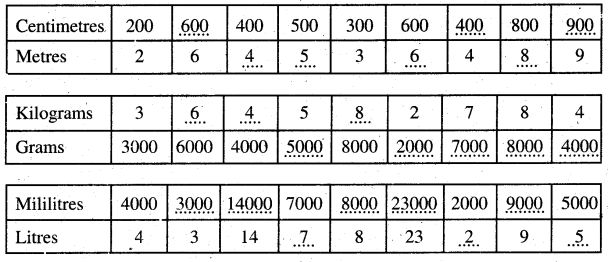# PSEB 5th Class Maths Solutions Chapter 6 Measurement Intext Questions

Punjab State Board PSEB 5th Class Maths Book Solutions Chapter 6 Measurement Intext Questions and Answers.

## PSEB Solutions for Class 5 Maths Chapter 6 Measurement Intext Questions

Page No. 130

Question 1.
How many pieces of 2 m can be cut from a 30 m long rope? How many times will you cut the rope?
Solution:
Length of rope = 30 m
Length of each piece = 2 m
No. of pieces that can be cut = 30 ÷ 2 = 15
No. of times we will cut the rope = 15 – 1 = 14Question 2.
Observe the following table and fill ups :Solution: